Question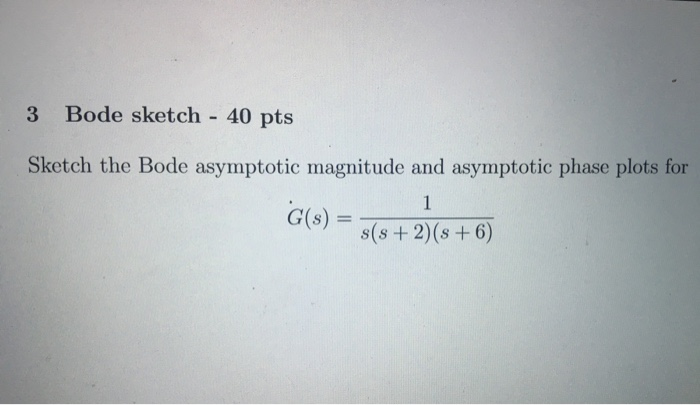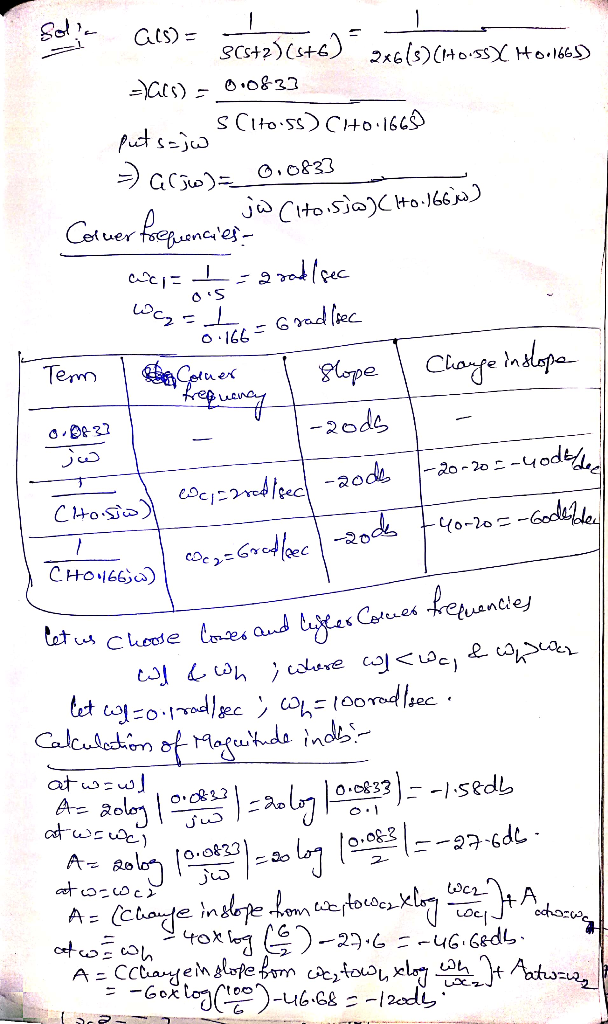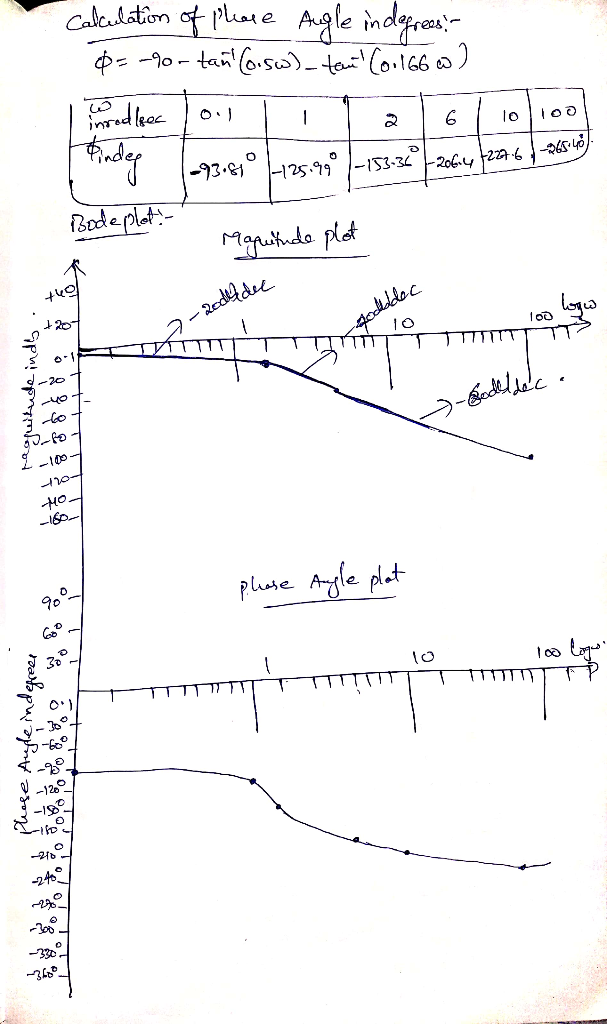#### Earn Coins

Coins can be redeemed for fabulous gifts.

Similar Homework Help Questions
• ### Problem 5: For the following transfer functions, sketch the bode asymptotic magnitude and phase p...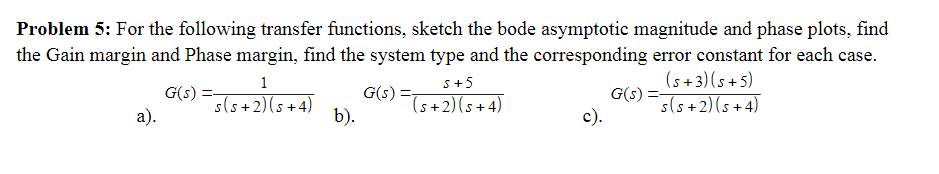Problem 5: For the following transfer functions, sketch the bode asymptotic magnitude and phase plots, find the Gain margin and Phase margin, find the system type and the corresponding error constant for each case. G(A) (s +3)(s +5) s(s +2) (s+4) S+5 2)b). Problem 5: For the following transfer functions, sketch the bode asymptotic magnitude and phase plots, find the Gain margin and Phase margin, find the system type and the corresponding error constant for each case. G(A) (s +3)(s...

• ### Sketch the Bode magnitude and phase plots for the following transfer function: G(s)=- a fimction: G(9)=...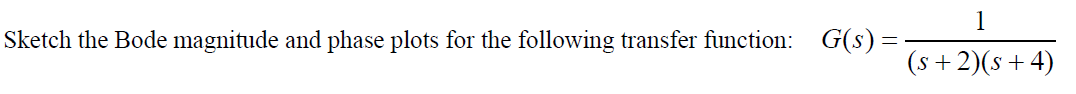Sketch the Bode magnitude and phase plots for the following transfer function: G(s)=- a fimction: G(9)= (s+2016+4) (s + 2)(+4)

• ### Sketch the approximate Bode magnitude and phase plots for the following transfer functions by hand. a....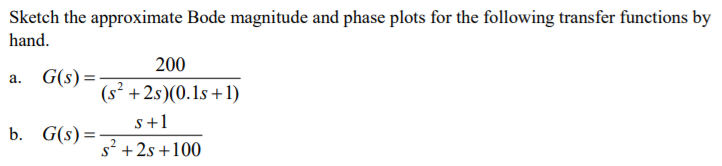Sketch the approximate Bode magnitude and phase plots for the following transfer functions by hand. a. G(s) b. G(s)- 200 (s2 +2s)(0.1s +1) s+1 s2 +2s +100

• ### For the following transfer function, sketch approximate straight-line Bode plots, including magnitude and phase plots. Show...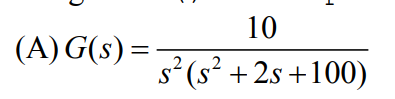For the following transfer function, sketch approximate straight-line Bode plots, including magnitude and phase plots. Show all steps clearly 10 4 (A)G(s)-7 s (s 2s +100)

• ### For the following closed-loop transfer functions, sketch the bode plots (magnitude and phase), id...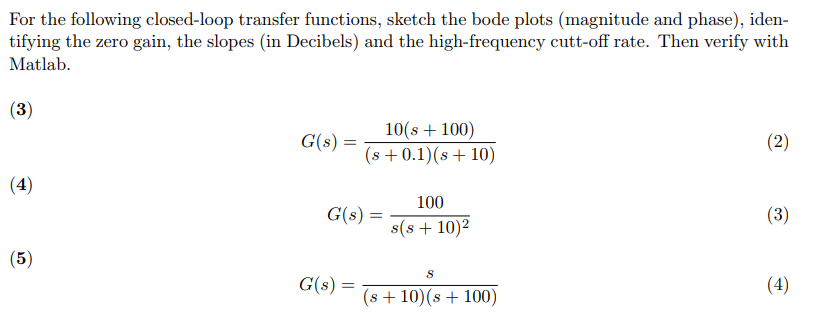For the following closed-loop transfer functions, sketch the bode plots (magnitude and phase), iden- tifying the zero gain, the slopes (in Decibels) and the high-frequency cutt-off rate. Then verify with Matlab C()101 100) s 0.1) (s 10) 100 s(s +10)2 G(s) = (56) G(s) = s+10(s+100) For the following closed-loop transfer functions, sketch the bode plots (magnitude and phase), iden- tifying the zero gain, the slopes (in Decibels) and the high-frequency cutt-off rate. Then verify with Matlab C()101 100) s...

• ### Bode Plots Sketch the Bode plot magnitude and phase for each of the three open-loop transfer...

Bode Plots Sketch the Bode plot magnitude and phase for each of the three open-loop transfer functions listed below. Verify your results using the bode m function in MATLAB.(a) $$G(s)=\frac{100}{s(0.1 s+1)(0.01 s+1)}$$(b) $$G(s)=\frac{1}{(s+1)^{2}\left(s^{2}+s+9\right)}$$(c) $$G(s)=\frac{16000 s}{(s+1)(s+100)\left(s^{2}+5 s+1600\right)}$$

• ### 2. Sketch the Bode straight line plots for magnitude and phase. a) H60) = a[i*3 (veja)...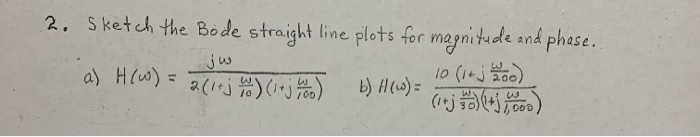2. Sketch the Bode straight line plots for magnitude and phase. a) H60) = a[i*3 (veja) b)H(s) og 10 (14 j k loo) (j +5.000) jw

• ### 2. [4pt] Sketch the Bode plots for the system below G(s) = S+ 2 s(s +...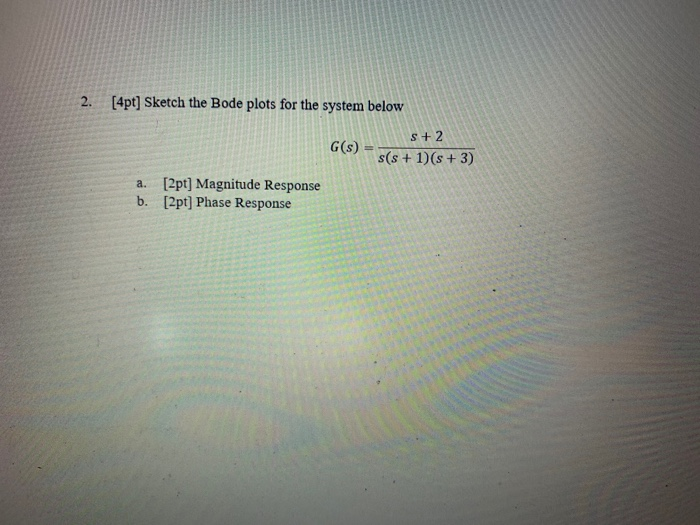2. [4pt] Sketch the Bode plots for the system below G(s) = S+ 2 s(s + 1)(s + 3) a. [2pt] Magnitude Response b. [2pt] Phase Response

• ### 1- For each transfer function below, sketch the Bode magnitude and phase plots, a) T(s) 3040S...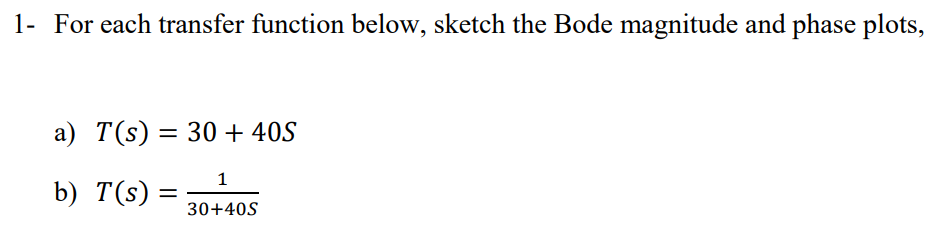1- For each transfer function below, sketch the Bode magnitude and phase plots, a) T(s) 3040S b) T(S) 30-405

• ### For the given system (s+25) P(s) s(s+1)(s+3000) 1. Sketch, by hand, the Bode asymptote plots for phase and magnitude. S...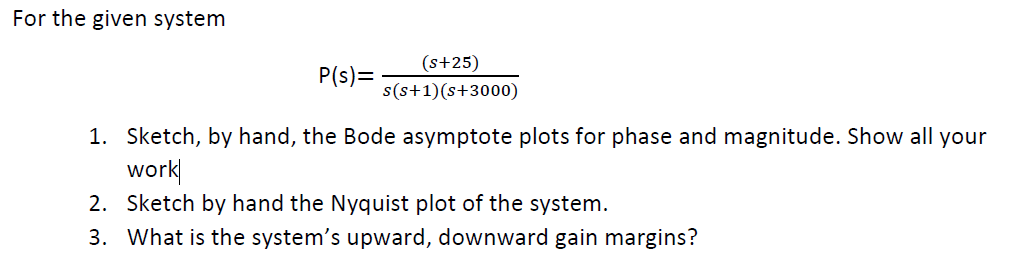For the given system (s+25) P(s) s(s+1)(s+3000) 1. Sketch, by hand, the Bode asymptote plots for phase and magnitude. Show all your work 2. Sketch by hand the Nyquist plot of the system 3. What is the system's upward, downward gain margins? For the given system (s+25) P(s) s(s+1)(s+3000) 1. Sketch, by hand, the Bode asymptote plots for phase and magnitude. Show all your work 2. Sketch by hand the Nyquist plot of the system 3. What is the system's...